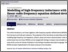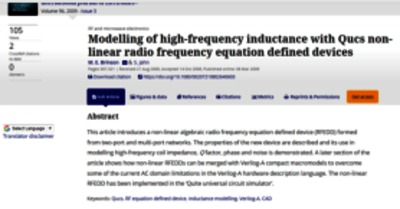Modelling of high-frequency inductance with Qucs non-linear radio frequency equation defined devices

Brinson, Mike and Jahn, Stefan (2009) Modelling of high-frequency inductance with Qucs non-linear radio frequency equation defined devices. International Journal of Electronics, 96 (3). pp. 307-321. ISSN 0020-7217Preview
Text
inductance.pdf - Cover Image

Abstract / Description

This article introduces a non-linear algebraic radio frequency equation defined device (RFEDD) formed from two-port and multi-port networks. The properties of the new device are described and its use in modelling high-frequency coil impedance, Q factor, phase and noise is demonstrated. A later section of the article shows how non-linear RFEDDs can be merged with Verilog-A compact macromodels to overcome some of the current AC domain limitations in the Verilog-A hardware description language. The non-linear RFEDD has been implemented in the ‘Quite universal circuit simulator’.

Item Type: Article Qucs; RF equation defined device; non-linear algebraic radio frequency equation defined device (RFEDD); inductance modelling; Verilog-A; CAD 600 Technology > 620 Engineering & allied operations School of Computing and Digital Media Mike Brinson 04 Nov 2019 10:40 04 Nov 2019 10:40 http://repository.londonmet.ac.uk/id/eprint/5268View Item